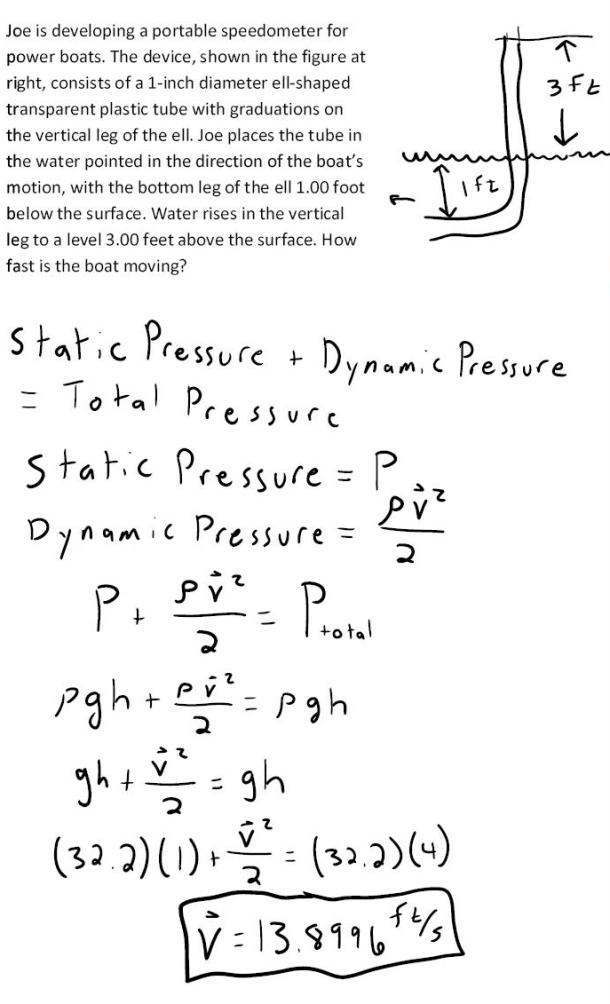fluid mechanics math problems engineering equations fluid mechanics basic mechanics school homework engineering math fluids formulas fluid mechanics problems fluid mechanics problem solutions to fluids problems full solution fluid mechanics engineering fluids problem solution fluid mechanics math problems engineering equations fluid mechanics basic mechanics school homework engineering math fluid mechanics formulas fluid problems fluid mechanics problem solutions to fluid mechanics problems full solution fluid mechanics engineering fluid mechanics problem solution
fluid mechanics math problems engineering equations fluid mechanics basic mechanics school homework engineering math fluids formulas fluid mechanics problems fluid mechanics problem solutions to fluids problems full solution fluid mechanics engineering fluids problem solution fluid mechanics math problems engineering equations fluid mechanics basic mechanics school homework engineering math fluid mechanics formulas fluid problems fluid mechanics problem solutions to fluid mechanics problems full solution fluid mechanics engineering fluid mechanics problem solution
Highalphabet Home Page fluid mechanics solutions fluids math solved Fluid Mechanics Page 1
Joe is developing a portable speedometer for power boats. The device, shown in the figure at right, consists of a 1-inch diameter ell-shaped transparent plastic tube with graduations on the vertical leg of the ell. Joe places the tube in the water pointed in the direction of the boat's motion, with the bottom leg of the ell 1.00 foot below the surface. Water rises in the vertical leg to a level of 3 feet above the surface. How fast is the boat moving?Joe is developing a portable speedometer for power boats. The device, shown in the figure at right, consists of a 1-inch diameter ell-shaped transparent plastic tube with graduations on the vertical leg of the ell. Joe places the tube in the water pointed in the direction of the boat's motion, with the bottom leg of the ell 1.00 foot below the surface. Water rises in the vertical leg to a level of 3 feet above the surface. How fast is the boat moving?